# Down the Π-holeAlthough a great deal is known about Π, not much of it is known by me, except that despite the irritating number of times I've been sagely informed that "Π ≡ 22 ÷ 7", it's is quite literally irrational (a mental state I will be compelled towards, if I hear that irritating non-fact just one more time). What that means, is that it can't be represented precisely by any fraction composed from an integral numerator & denominator. Since decimal numbers are just fractions in which the denominator is constrained to a power of ten, they won't suffice either; so it's just as well that the Indiana Pi Bill, didn't make it into law, otherwise people would be sagely informing me it equals 3.2, & I would end up scrawling its interminable digits, using a wax crayon gripped between my toes, on the walls of my cell.

Calculating Π to an arbitrary number of digits may seem like an odd pursuit, & I can't quite recall the reason why I started (perhaps "Police Academy 7" was on TV; which ironically also appears to be an endless series), but because of the huge variety of methods, it has turned into a rich seam to mine, requiring the calculation of square-roots to arbitrary precision, & the factorials of large integers. These formulae represent a small fraction of those available, but are representative of the various approaches to the problem.

Pi-formulae implemented in package "factory".
Name Order of
Convergence
Rate of
Convergence
Formula
Rabinowitz-Wagon Linear 10-3 / 10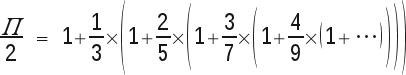Gosper Linear 10-1.11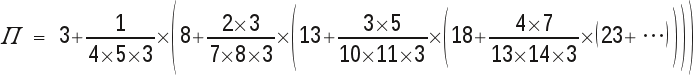Bellard Linear 2-10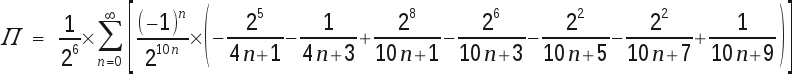Bailey-Borwein-Plouffe-type Linear 2-16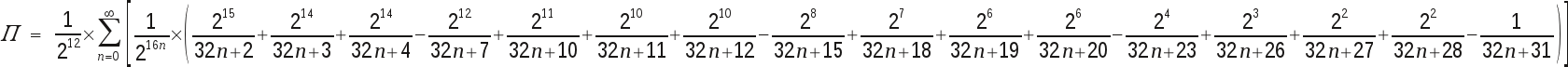Ramanujan Linear 10-7.9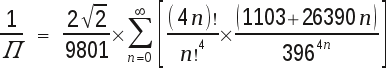Chudnovsky Linear 10-14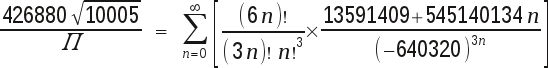Borwein Linear 10-50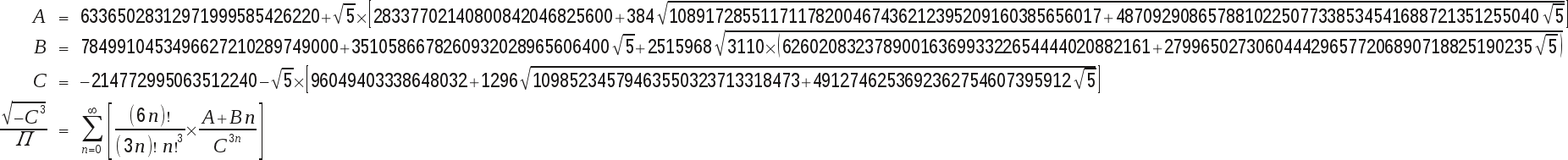Brent-Salamin,
()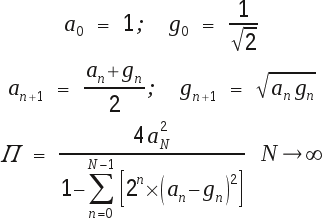Series:
Rabinowitz-Wagon & Gosper:

These are continued fractions, which have been implemented as spigot-algorithms. This conveniently only involves machine-width integer-arithmetic, whereas the remaining algorithms use unbounded rational arithmetic.

Bellard & Bailey-Borwein-Plouffe-type (BBP):

Many instances of this type of series, permit determination of specific digits of Pi (when operating in a numeral-system whose base matches the convergence-rate), without determining preceding digits; though that's not the manner in which it's implemented here.

Ramanujan & Chudnovsky:

These series are a little trickier, since they involve the calculation of the principal square-root of a constant integer. This doesn't seem onerous, until one realises that this will be required to an equivalent arbitrary precision. They also involve factorials of potentially large integers.

Borwein:

The series I've used (one discovered in 1993, from many attributed to the same source) is structurally very similar to the Chudnovsky brothers' formula, but with a much faster convergence, yielding fifty digits per term.

Iterations:
Brent-Salamin:

The series above, have all exhibited linear order of convergence. This means that for each term in the series, the approximation to Π is improved by a constant number of digits. The rate of convergence, (which governs the value of this constant) depends on the specific series. This iteration, in contrast, has a quadratic order of convergence, which means that the number of correct digits in the approximation doubles with each iteration.

## Testing

My usual test-platform was used. (Many of the algorithms parallelize effectively, but lacking the hardware for a useful analysis, I've limited testing to just one core).

## Results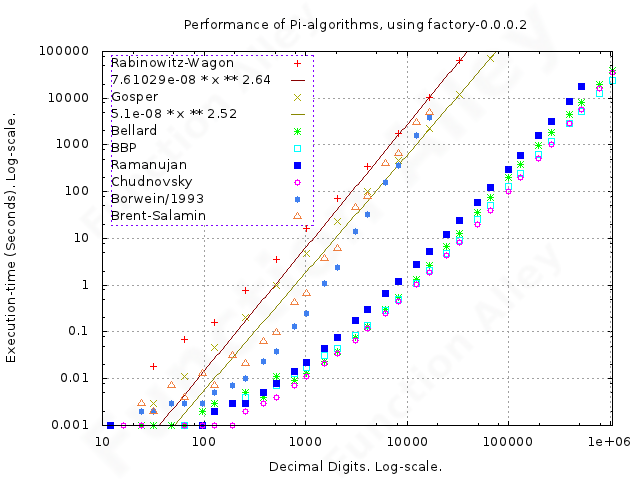As noted when finding square-roots by expansion of their Taylor-series, most of these algorithms are sensitive to the speed at which one can sum rational numbers. Whilst summation is normally a relatively inexpensive operation, it comes as a rude awakening that it isn't when operating on rational numbers, since it typically involves folding cross-multiplication over the list. The previously employed performance-enhancing strategy can now be seen to be a ghastly compromise between time & space, when applied to the long sequences of rational numbers (particularly if they haven't been reduced to that representation with minimal numerator & denominator) encountered here. This was countered by splitting the list of rational numbers into chunks, of a length chosen to balance the greed for speed with that for the heap.

I've fitted polynomials to the data which exhibit some clear trend, so that one can estimate their complexity-class. Because of the log-log plot, these polynomials appear as straight lines.

Most algorithms appear to have approximately O(n2) time-complexity. One may have predicted this for the spigot-algorithms, since they're implemented using a table, both of whose dimensions are proportional to the required precision, & each calculation is just fixed-width integer-arithmetic. Both the Borwein formula & the Brent-Salamin iteration look a shade more exponential than polynomial; though clarity is sadly lacking, & it may equally be that their gargantuan memory-requirement influences computation-time more than the number of steps required.

Because the quadratic order of convergence of the Brent-Salamin iteration, results in the precision doubling with each iteration, a staggering of the results is apparent; both the execution-time & memory-requirement pulse above the underlying trend, between those precision-requirements which exactly match the calculated precision, & where in consequence, no excess precision has been calculated. This unwelcome behaviour can be also observed to a lesser extent in other algorithms.

The two spigot-algorithms, performed worst over the precision-range tested, though it looks likely that both the Borwein formula & Brent-Salamin iteration, will result in worse performance at slightly higher precisions. The remaining algorithms performed broadly similarly, but with the "base-65536"-version of the Bailey-Borwein-Plouffe-type formulae, marginally the fastest.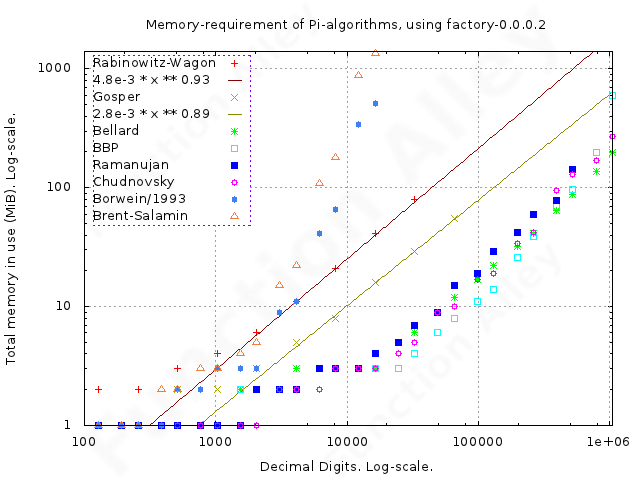The memory-requirements of all algorithms have approximately O(n) space-complexity, but the Borwein formula & Brent-Salamin iteration stood-out again, being closer to O(n3), rapidly limiting my ability to test further on the available hardware.

## Conclusions

None of these implementations are competitive with record-breaking implementations; nobody raises even one eyebrow until the first trillion digits have whistled by. Broadly speaking, one might expect an application coded in Haskell, to run at only half the speed of a similar algorithm coded in C++; see the Code-shootout. In addition, I have to admit the possibility that my implementation stinks, but if this is so, then all my implementations are similarly blighted. This may be so (since it's regrettably easy, when using rational arithmetic to accidentally use a fraction with a huge numerator & denominator, where a much simpler fractional-representation, with adequate precision, is also available), but I've found no smoking gun yet.

Despite the poor overall absolute performance of all the implementations, the relatively poor performance of the Brent-Salamin iteration, was a surprise. Its quadratic order of convergence suggested superiority over the other algorithms, but regrettably each iteration demands vastly more resources than the previous.